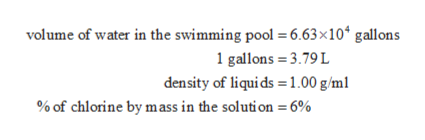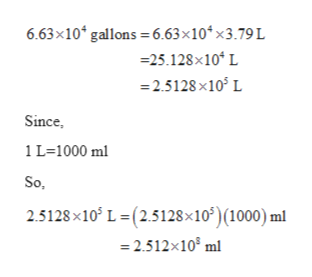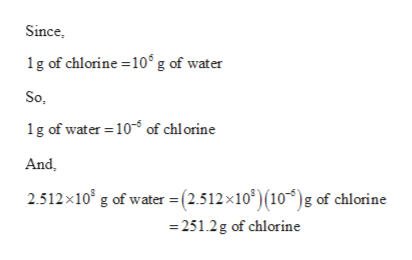# Chlorine is used to disinfect swimming pools. The accepted concentration for this purpose is 1 ppm chlorine or 1 g of chlorine per million g of water. Calculate the volume of a chlorine solution (in milliliters) a homeowner should add to her swimming pool to achieve 1.00 ppm chlorine if the solution contains 6.00 perce chlorine by mass and there are 6.63 ×104 gallons of water in the pool. (1 gallon = 3.79 L; density of liquids = 1.00 g/mL.)

Question
465 views

Chlorine is used to disinfect swimming pools. The accepted concentration for this purpose is 1 ppm chlorine or 1 g of chlorine per million g of water. Calculate the volume of a chlorine solution (in milliliters) a homeowner should add to her swimming pool to achieve 1.00 ppm chlorine if the solution contains 6.00 perce chlorine by mass and there are 6.63 ×104 gallons of water in the pool. (1 gallon = 3.79 L; density of liquids = 1.00 g/mL.)

check_circle

Step 1

The values are given as follows:help_outlineImage Transcriptionclosevolume of water in the swimming pool =6.63 x104 gallons 1 gallons 3.79L density of liquids 1.00 g/m % of chlorine by mass in the soluti on 6% fullscreen
Step 2

The concentration of chlorine consume is 1 g of Cl in 106 g of water.

Total volume of water in the swimming pool can be calculated as follows:help_outlineImage Transcriptionclose6.63x10 gallons 6.63x10 x3.79 L 25.128x10 L 2.5128 x10 L Since 1 L-1000 ml So. 2.5128x10 L-(2.5128x10)(1000) ml 2.512x10 ml fullscreen
Step 3

Density of water is 1 g/ml so, mass of water ...help_outlineImage TranscriptioncloseSince 1g of chlorine 10g of water So 1g of water 10 of chlorine And g of water = (2.512x10) (10)g of chlorine 251.2g of chlorine 2.512x10 fullscreen

### Want to see the full answer?

See Solution

#### Want to see this answer and more?

Solutions are written by subject experts who are available 24/7. Questions are typically answered within 1 hour.*

See Solution
*Response times may vary by subject and question.
Tagged in

### Chemistry